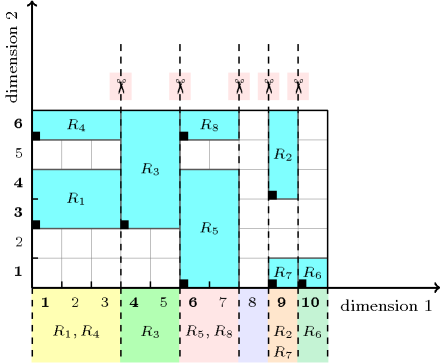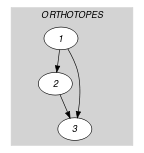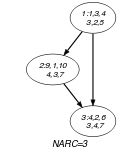## 5.119. diffn_column

Origin

CHIP: option guillotine cut (column) of $\mathrm{𝚍𝚒𝚏𝚏𝚗}$.

Constraint

$\mathrm{𝚍𝚒𝚏𝚏𝚗}_\mathrm{𝚌𝚘𝚕𝚞𝚖𝚗}\left(\mathrm{𝙾𝚁𝚃𝙷𝙾𝚃𝙾𝙿𝙴𝚂},\mathrm{𝙳𝙸𝙼}\right)$

Type
 $\mathrm{𝙾𝚁𝚃𝙷𝙾𝚃𝙾𝙿𝙴}$ $\mathrm{𝚌𝚘𝚕𝚕𝚎𝚌𝚝𝚒𝚘𝚗}\left(\mathrm{𝚘𝚛𝚒}-\mathrm{𝚍𝚟𝚊𝚛},\mathrm{𝚜𝚒𝚣}-\mathrm{𝚍𝚟𝚊𝚛},\mathrm{𝚎𝚗𝚍}-\mathrm{𝚍𝚟𝚊𝚛}\right)$
Arguments
 $\mathrm{𝙾𝚁𝚃𝙷𝙾𝚃𝙾𝙿𝙴𝚂}$ $\mathrm{𝚌𝚘𝚕𝚕𝚎𝚌𝚝𝚒𝚘𝚗}\left(\mathrm{𝚘𝚛𝚝𝚑}-\mathrm{𝙾𝚁𝚃𝙷𝙾𝚃𝙾𝙿𝙴}\right)$ $\mathrm{𝙳𝙸𝙼}$ $\mathrm{𝚒𝚗𝚝}$
Restrictions
 $|\mathrm{𝙾𝚁𝚃𝙷𝙾𝚃𝙾𝙿𝙴}|>0$ $\mathrm{𝚛𝚎𝚚𝚞𝚒𝚛𝚎}_\mathrm{𝚊𝚝}_\mathrm{𝚕𝚎𝚊𝚜𝚝}$$\left(2,\mathrm{𝙾𝚁𝚃𝙷𝙾𝚃𝙾𝙿𝙴},\left[\mathrm{𝚘𝚛𝚒},\mathrm{𝚜𝚒𝚣},\mathrm{𝚎𝚗𝚍}\right]\right)$ $\mathrm{𝙾𝚁𝚃𝙷𝙾𝚃𝙾𝙿𝙴}.\mathrm{𝚜𝚒𝚣}\ge 0$ $\mathrm{𝙾𝚁𝚃𝙷𝙾𝚃𝙾𝙿𝙴}.\mathrm{𝚘𝚛𝚒}\le \mathrm{𝙾𝚁𝚃𝙷𝙾𝚃𝙾𝙿𝙴}.\mathrm{𝚎𝚗𝚍}$ $\mathrm{𝚛𝚎𝚚𝚞𝚒𝚛𝚎𝚍}$$\left(\mathrm{𝙾𝚁𝚃𝙷𝙾𝚃𝙾𝙿𝙴𝚂},\mathrm{𝚘𝚛𝚝𝚑}\right)$ $\mathrm{𝚜𝚊𝚖𝚎}_\mathrm{𝚜𝚒𝚣𝚎}$$\left(\mathrm{𝙾𝚁𝚃𝙷𝙾𝚃𝙾𝙿𝙴𝚂},\mathrm{𝚘𝚛𝚝𝚑}\right)$ $\mathrm{𝙳𝙸𝙼}>0$ $\mathrm{𝙳𝙸𝙼}\le |\mathrm{𝙾𝚁𝚃𝙷𝙾𝚃𝙾𝙿𝙴}|$ $\mathrm{𝚍𝚒𝚏𝚏𝚗}$$\left(\mathrm{𝙾𝚁𝚃𝙷𝙾𝚃𝙾𝙿𝙴𝚂}\right)$
Purpose

Extension of the generalised multi-dimensional non-overlapping diffn constraint. Holds if, for each pair of orthotopes $\left({O}_{1},{O}_{2}\right)$ the following conditions hold:

• ${O}_{1}$ and ${O}_{2}$ do not overlap. Two orthotopes do not overlap if one of the orthotopes has zero size or if there exists at least one dimension where their projections do not overlap.

• Let ${P}_{1}$ and ${P}_{2}$ respectively denote the projections of ${O}_{1}$ and ${O}_{2}$ onto dimension $\mathrm{𝙳𝙸𝙼}$. If ${P}_{1}$ and ${P}_{2}$ overlap then the size of their intersection is equal to the size of ${O}_{1}$ in dimension $\mathrm{𝙳𝙸𝙼}$, as well as to the size of ${O}_{2}$ in dimension $\mathrm{𝙳𝙸𝙼}$.

Example
$\left(\begin{array}{c}〈\begin{array}{c}\mathrm{𝚘𝚛𝚝𝚑}-〈\mathrm{𝚘𝚛𝚒}-1\mathrm{𝚜𝚒𝚣}-3\mathrm{𝚎𝚗𝚍}-4,\mathrm{𝚘𝚛𝚒}-3\mathrm{𝚜𝚒𝚣}-2\mathrm{𝚎𝚗𝚍}-5〉,\hfill \\ \mathrm{𝚘𝚛𝚝𝚑}-〈\begin{array}{ccc}\mathrm{𝚘𝚛𝚒}-9\hfill & \mathrm{𝚜𝚒𝚣}-1\hfill & \mathrm{𝚎𝚗𝚍}-10,\hfill \\ \mathrm{𝚘𝚛𝚒}-4\hfill & \mathrm{𝚜𝚒𝚣}-3\hfill & \mathrm{𝚎𝚗𝚍}-7\hfill \end{array}〉,\hfill \\ \mathrm{𝚘𝚛𝚝𝚑}-〈\mathrm{𝚘𝚛𝚒}-4\mathrm{𝚜𝚒𝚣}-2\mathrm{𝚎𝚗𝚍}-6,\mathrm{𝚘𝚛𝚒}-3\mathrm{𝚜𝚒𝚣}-4\mathrm{𝚎𝚗𝚍}-7〉,\hfill \\ \mathrm{𝚘𝚛𝚝𝚑}-〈\mathrm{𝚘𝚛𝚒}-1\mathrm{𝚜𝚒𝚣}-3\mathrm{𝚎𝚗𝚍}-4,\mathrm{𝚘𝚛𝚒}-6\mathrm{𝚜𝚒𝚣}-1\mathrm{𝚎𝚗𝚍}-7〉,\hfill \\ \mathrm{𝚘𝚛𝚝𝚑}-〈\mathrm{𝚘𝚛𝚒}-6\mathrm{𝚜𝚒𝚣}-2\mathrm{𝚎𝚗𝚍}-8,\mathrm{𝚘𝚛𝚒}-1\mathrm{𝚜𝚒𝚣}-4\mathrm{𝚎𝚗𝚍}-5〉,\hfill \\ \mathrm{𝚘𝚛𝚝𝚑}-〈\begin{array}{ccc}\mathrm{𝚘𝚛𝚒}-10\hfill & \mathrm{𝚜𝚒𝚣}-1\hfill & \mathrm{𝚎𝚗𝚍}-11,\hfill \\ \mathrm{𝚘𝚛𝚒}-1\hfill & \mathrm{𝚜𝚒𝚣}-1\hfill & \mathrm{𝚎𝚗𝚍}-2\hfill \end{array}〉,\hfill \\ \mathrm{𝚘𝚛𝚝𝚑}-〈\begin{array}{ccc}\mathrm{𝚘𝚛𝚒}-9\hfill & \mathrm{𝚜𝚒𝚣}-1\hfill & \mathrm{𝚎𝚗𝚍}-10,\hfill \\ \mathrm{𝚘𝚛𝚒}-1\hfill & \mathrm{𝚜𝚒𝚣}-1\hfill & \mathrm{𝚎𝚗𝚍}-2\hfill \end{array}〉,\hfill \\ \mathrm{𝚘𝚛𝚝𝚑}-〈\mathrm{𝚘𝚛𝚒}-6\mathrm{𝚜𝚒𝚣}-2\mathrm{𝚎𝚗𝚍}-8,\mathrm{𝚘𝚛𝚒}-6\mathrm{𝚜𝚒𝚣}-1\mathrm{𝚎𝚗𝚍}-7〉\hfill \end{array}〉,1\hfill \end{array}\right)$

Figure 5.119.1 represents the respective position of the eight rectangles of the example. The coordinates of the leftmost lowest corner of each rectangle are stressed in bold. The $\mathrm{𝚍𝚒𝚏𝚏𝚗}_\mathrm{𝚌𝚘𝚕𝚞𝚖𝚗}$ constraint holds since (1) the eight rectangles do not overlap and since (2) when their projection onto dimension $\mathrm{𝙳𝙸𝙼}=1$ overlap the size of their intersection is equal to the size of the corresponding rectangles in dimension $\mathrm{𝙳𝙸𝙼}=1$.

##### Figure 5.119.1. Illustration of the Example slot: eight non-overlapping rectangles such that, for each pair of rectangles ${R}_{i}$, ${R}_{j}$$\left(1\le i, if the projections onto dimension 1 of rectangles ${R}_{i}$ and ${R}_{j}$ intersect then the size of their intersection is equal to the size of ${R}_{i}$ in dimension 1 and to the size of ${R}_{j}$ in dimension 1 (i.e. complete vertical strips along the border of any rectangle can be cut without crossing any rectangle)Typical
 $|\mathrm{𝙾𝚁𝚃𝙷𝙾𝚃𝙾𝙿𝙴}|>1$ $\mathrm{𝙾𝚁𝚃𝙷𝙾𝚃𝙾𝙿𝙴}.\mathrm{𝚜𝚒𝚣}>0$ $|\mathrm{𝙾𝚁𝚃𝙷𝙾𝚃𝙾𝙿𝙴𝚂}|>1$
Symmetries
• Items of $\mathrm{𝙾𝚁𝚃𝙷𝙾𝚃𝙾𝙿𝙴𝚂}$ are permutable.

• One and the same constant can be added to the $\mathrm{𝚘𝚛𝚒}$ and $\mathrm{𝚎𝚗𝚍}$ attributes of all items of $\mathrm{𝙾𝚁𝚃𝙷𝙾𝚃𝙾𝙿𝙴𝚂}.\mathrm{𝚘𝚛𝚝𝚑}$.

Arg. properties

Contractible wrt. $\mathrm{𝙾𝚁𝚃𝙷𝙾𝚃𝙾𝙿𝙴𝚂}$.

Keywords
Arc input(s)

$\mathrm{𝙾𝚁𝚃𝙷𝙾𝚃𝙾𝙿𝙴𝚂}$

Arc generator
$\mathrm{𝐶𝐿𝐼𝑄𝑈𝐸}$$\left(<\right)↦\mathrm{𝚌𝚘𝚕𝚕𝚎𝚌𝚝𝚒𝚘𝚗}\left(\mathrm{𝚘𝚛𝚝𝚑𝚘𝚝𝚘𝚙𝚎𝚜}\mathtt{1},\mathrm{𝚘𝚛𝚝𝚑𝚘𝚝𝚘𝚙𝚎𝚜}\mathtt{2}\right)$

Arc arity
Arc constraint(s)
$\mathrm{𝚝𝚠𝚘}_\mathrm{𝚘𝚛𝚝𝚑}_\mathrm{𝚌𝚘𝚕𝚞𝚖𝚗}$$\left(\mathrm{𝚘𝚛𝚝𝚑𝚘𝚝𝚘𝚙𝚎𝚜}\mathtt{1}.\mathrm{𝚘𝚛𝚝𝚑},\mathrm{𝚘𝚛𝚝𝚑𝚘𝚝𝚘𝚙𝚎𝚜}\mathtt{2}.\mathrm{𝚘𝚛𝚝𝚑},\mathrm{𝙳𝙸𝙼}\right)$
Graph property(ies)
$\mathrm{𝐍𝐀𝐑𝐂}$$=|\mathrm{𝙾𝚁𝚃𝙷𝙾𝚃𝙾𝙿𝙴𝚂}|*\left(|\mathrm{𝙾𝚁𝚃𝙷𝙾𝚃𝙾𝙿𝙴𝚂}|-1\right)/2$

Graph model

Since showing all items produces too big graphs, parts (A) and (B) of Figure 5.119.2 respectively show the initial and final graph associated with the first three items of the Example slot. Since we use the $\mathrm{𝐍𝐀𝐑𝐂}$ graph property, the arcs of the final graph are stressed in bold.

##### Figure 5.119.2. Initial and final graph of the $\mathrm{𝚍𝚒𝚏𝚏𝚗}_\mathrm{𝚌𝚘𝚕𝚞𝚖𝚗}$ constraint(a) (b)